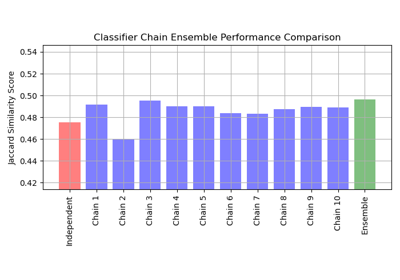# sklearn.metrics.jaccard_score¶

sklearn.metrics.jaccard_score(y_true, y_pred, *, labels=None, pos_label=1, average='binary', sample_weight=None, zero_division='warn')[source]

Jaccard similarity coefficient score.

The Jaccard index , or Jaccard similarity coefficient, defined as the size of the intersection divided by the size of the union of two label sets, is used to compare set of predicted labels for a sample to the corresponding set of labels in y_true.

Read more in the User Guide.

Parameters:
y_true1d array-like, or label indicator array / sparse matrix

Ground truth (correct) labels.

y_pred1d array-like, or label indicator array / sparse matrix

Predicted labels, as returned by a classifier.

labelsarray-like of shape (n_classes,), default=None

The set of labels to include when average != 'binary', and their order if average is None. Labels present in the data can be excluded, for example to calculate a multiclass average ignoring a majority negative class, while labels not present in the data will result in 0 components in a macro average. For multilabel targets, labels are column indices. By default, all labels in y_true and y_pred are used in sorted order.

pos_labelstr or int, default=1

The class to report if average='binary' and the data is binary. If the data are multiclass or multilabel, this will be ignored; setting labels=[pos_label] and average != 'binary' will report scores for that label only.

average{‘micro’, ‘macro’, ‘samples’, ‘weighted’, ‘binary’} or None, default=’binary’

If None, the scores for each class are returned. Otherwise, this determines the type of averaging performed on the data:

'binary':

Only report results for the class specified by pos_label. This is applicable only if targets (y_{true,pred}) are binary.

'micro':

Calculate metrics globally by counting the total true positives, false negatives and false positives.

'macro':

Calculate metrics for each label, and find their unweighted mean. This does not take label imbalance into account.

'weighted':

Calculate metrics for each label, and find their average, weighted by support (the number of true instances for each label). This alters ‘macro’ to account for label imbalance.

'samples':

Calculate metrics for each instance, and find their average (only meaningful for multilabel classification).

sample_weightarray-like of shape (n_samples,), default=None

Sample weights.

zero_division“warn”, {0.0, 1.0}, default=”warn”

Sets the value to return when there is a zero division, i.e. when there there are no negative values in predictions and labels. If set to “warn”, this acts like 0, but a warning is also raised.

Returns:
scorefloat or ndarray of shape (n_unique_labels,), dtype=np.float64

The Jaccard score. When average is not None, a single scalar is returned.

accuracy_score

Function for calculating the accuracy score.

f1_score

Function for calculating the F1 score.

multilabel_confusion_matrix

Function for computing a confusion matrix for each class or sample.

Notes

jaccard_score may be a poor metric if there are no positives for some samples or classes. Jaccard is undefined if there are no true or predicted labels, and our implementation will return a score of 0 with a warning.

References

Examples

>>> import numpy as np
>>> from sklearn.metrics import jaccard_score
>>> y_true = np.array([[0, 1, 1],
...                    [1, 1, 0]])
>>> y_pred = np.array([[1, 1, 1],
...                    [1, 0, 0]])


In the binary case:

>>> jaccard_score(y_true, y_pred)
0.6666...


In the 2D comparison case (e.g. image similarity):

>>> jaccard_score(y_true, y_pred, average="micro")
0.6


In the multilabel case:

>>> jaccard_score(y_true, y_pred, average='samples')
0.5833...
>>> jaccard_score(y_true, y_pred, average='macro')
0.6666...
>>> jaccard_score(y_true, y_pred, average=None)
array([0.5, 0.5, 1. ])


In the multiclass case:

>>> y_pred = [0, 2, 1, 2]
>>> y_true = [0, 1, 2, 2]
>>> jaccard_score(y_true, y_pred, average=None)
array([1. , 0. , 0.33...])


## Examples using sklearn.metrics.jaccard_score¶Classifier Chain

Classifier Chain